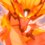# Finding Euler

$\sum_{n=1}^{\infty} \dfrac{\overline{H_{n}}}{n^q} = \zeta(q) \log 2 - \dfrac{q}{2} \zeta(q+1) + \sum_{k=1}^{q} \eta(k) \eta(q-k+1)$

Prove the equation above.

Notation : $\displaystyle \overline{H_{n}} = \sum_{k=1}^n \frac{(-1)^{k-1}}{k}$ denotes the Alternating Harmonic Number.

$\displaystyle \zeta(s) = \sum_{k=1}^{\infty} \dfrac{1}{k^s}$ denotes the Riemann Zeta Function.

$\displaystyle \eta(s) = \sum_{k=1}^{\infty} \dfrac{(-1)^{k-1}}{k^s}$ denotes the Dirchlet Eta Function.Note by Ishan Singh
4 years, 9 months ago

This discussion board is a place to discuss our Daily Challenges and the math and science related to those challenges. Explanations are more than just a solution — they should explain the steps and thinking strategies that you used to obtain the solution. Comments should further the discussion of math and science.

When posting on Brilliant:

• Use the emojis to react to an explanation, whether you're congratulating a job well done , or just really confused .
• Ask specific questions about the challenge or the steps in somebody's explanation. Well-posed questions can add a lot to the discussion, but posting "I don't understand!" doesn't help anyone.
• Try to contribute something new to the discussion, whether it is an extension, generalization or other idea related to the challenge.

MarkdownAppears as
*italics* or _italics_ italics
**bold** or __bold__ bold
- bulleted- list
• bulleted
• list
1. numbered2. list
1. numbered
2. list
Note: you must add a full line of space before and after lists for them to show up correctly
paragraph 1paragraph 2

paragraph 1

paragraph 2

[example link](https://brilliant.org)example link
> This is a quote
This is a quote
    # I indented these lines
# 4 spaces, and now they show
# up as a code block.

print "hello world"
# I indented these lines
# 4 spaces, and now they show
# up as a code block.

print "hello world"
MathAppears as
Remember to wrap math in $$ ... $$ or $ ... $ to ensure proper formatting.
2 \times 3 $2 \times 3$
2^{34} $2^{34}$
a_{i-1} $a_{i-1}$
\frac{2}{3} $\frac{2}{3}$
\sqrt{2} $\sqrt{2}$
\sum_{i=1}^3 $\sum_{i=1}^3$
\sin \theta $\sin \theta$
\boxed{123} $\boxed{123}$

Sort by:

Since $\displaystyle \overline{H_n} = \sum_{k=1}^{n}\frac{(-1)^{k-1}}{k}=\int_{0}^{1} \frac{1-(-x)^n}{1+x}dx$ ,

Summing both sides we obtain $\displaystyle \sum_{n=1}^{\infty} \frac{\overline{H_n}}{n^q} = \int_{0}^{1}\frac{\zeta(q)}{1+x}dx - \int_{0}^{1}\frac{Li_q(-x)}{1+x}dx=\zeta(q)\ln 2 -\underbrace{\int_{0}^{1}\frac{Li_q(-x)}{1+x}dx}_{\color{#D61F06}{I}}$

Now applying IBP we have, $\displaystyle \color{#D61F06}{I} = \int_{0}^{1}\frac{Li_q(-x)}{1+x}dx = [Li_q(-x)\ln(1+x)]_{0}^{1}+\int_{0}^{1} \frac{Li_{q-1}(-x)\ln(1+x)}{x}dx$

Since $\displaystyle Li_q(-1)=-\eta(q),\eta(1)=\ln 2$ and applying IBP again,

$\displaystyle \color{#D61F06}{I} = -\eta(q)\eta(1)-[Li_{q-1}(-x)Li_2(-x)]_{0}^{1} - \int_{0}^{1} \frac{Li_{q-2}(-x)Li_2(-x)}{x}dx = -\eta(q)\eta(1)-\eta(q-1)\eta(2)+ \int_{0}^{1} \frac{Li_{q-2}(-x)Li_2(-x)}{x}dx$

Similarly applying IBP $p$ times , we will get

$\displaystyle \color{#D61F06}{I}=-\sum_{r=1}^{q}\eta(q-r+1)\eta(r) -\eta(q+1)$

Therefore , $\displaystyle \sum_{n=1}^{\infty} \frac{\overline{H_n}}{n^q}=\zeta(q)\ln2+\sum_{k=1}^{q}\eta(q-k+1)\eta(k)+\eta(q+1)$ for odd $q$

- 4 years, 9 months ago

Note that when you apply IBP, every time the sign of the integral changes. So your formula will regenerate the integral only if $q$ is odd. In other words, your formula is correct, when $q$ is odd. For instance, I have solved the regular Euler Sum here, in a similar way before.

- 4 years, 9 months ago

So I may assume that the result as quoted by you of this summation is for the even $q$ ?

- 4 years, 9 months ago

No, it is for all $q$, even or odd.

- 4 years, 9 months ago

There is another mistake in your formula, from what you have done, there should be a $(-1)^k$ inside the summation

- 4 years, 9 months ago

The formula for the alternate harmonic sum is fine .it's written (-x) and not x

- 4 years, 9 months ago

As I said, there is a sign error in the second red line, it should be $+ \int_{0}^{1} \dfrac{\operatorname{Li}_{q-2}(-x) \operatorname{Li}_2(-x)}{x} \mathrm{d}x$ and not $- \int_{0}^{1} \dfrac{\operatorname{Li}_{q-2}(-x) \operatorname{Li}_2(-x)}{x} \mathrm{d}x$ (even if we take (-x)).

- 4 years, 9 months ago

Thanks for that , tou said there should be a $(-1)^k$ in your summation. Summation reffers only to the integral representation of the alternate harmonic number. But that's fine. I said there is a $(-x)$ in that formulae

- 4 years, 9 months ago

I meant in the eta summation, there should be a $(-1)^k$ in the sum. Compare with original Euler Sum in my answer here.

- 4 years, 9 months ago

Also, there seems to be a sign error in the second red line, it should be + integral 0 to 1 ((Li)(q-2) (x) Li(2) (x))/x dx

- 4 years, 9 months ago